Question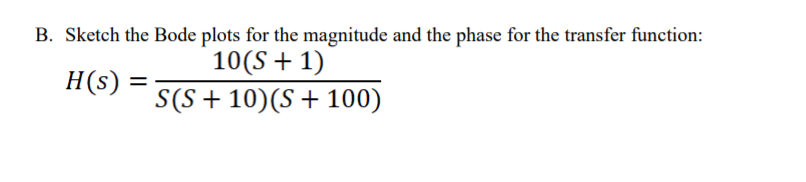Please plot on semi-log scale for both magnitude and phase separately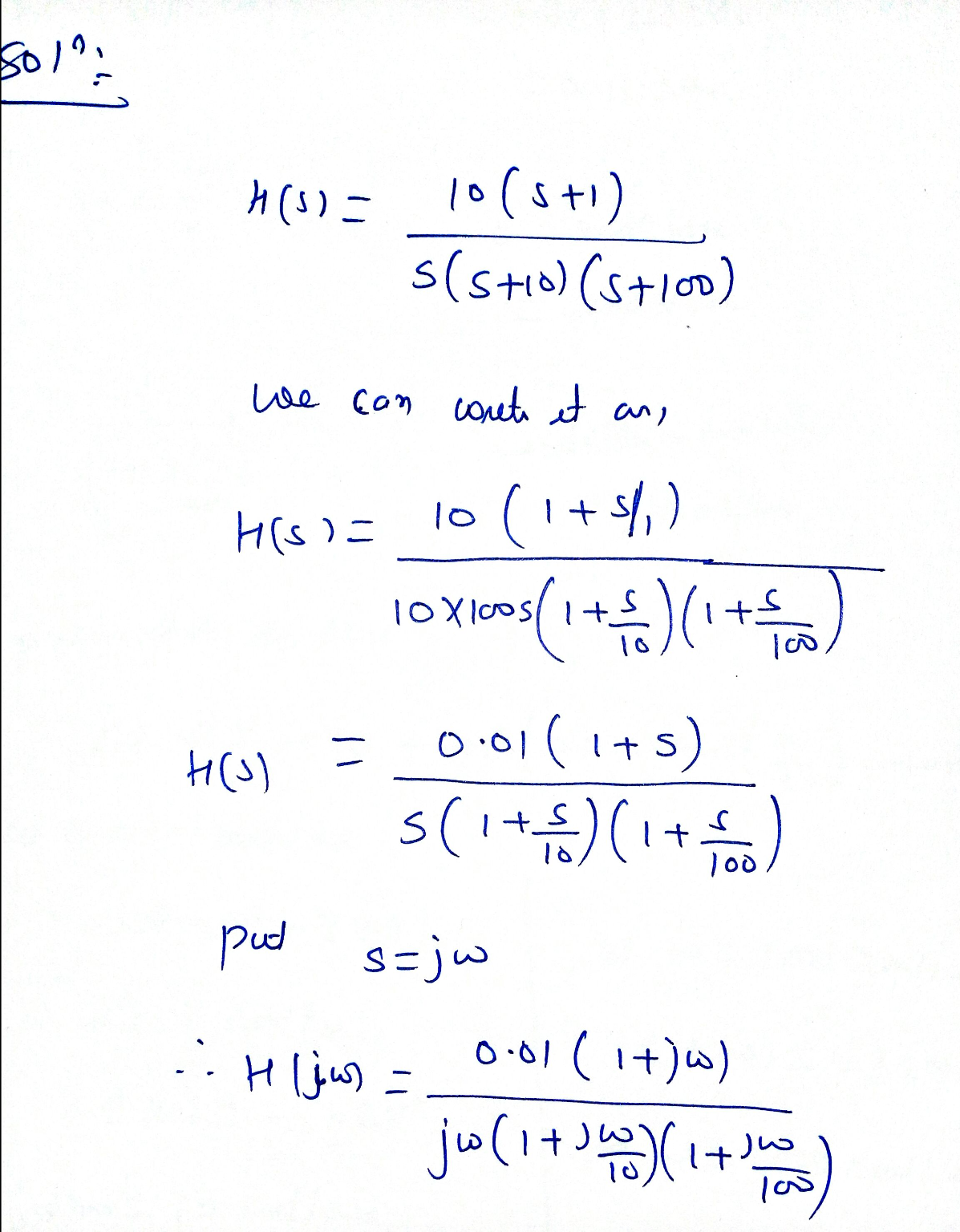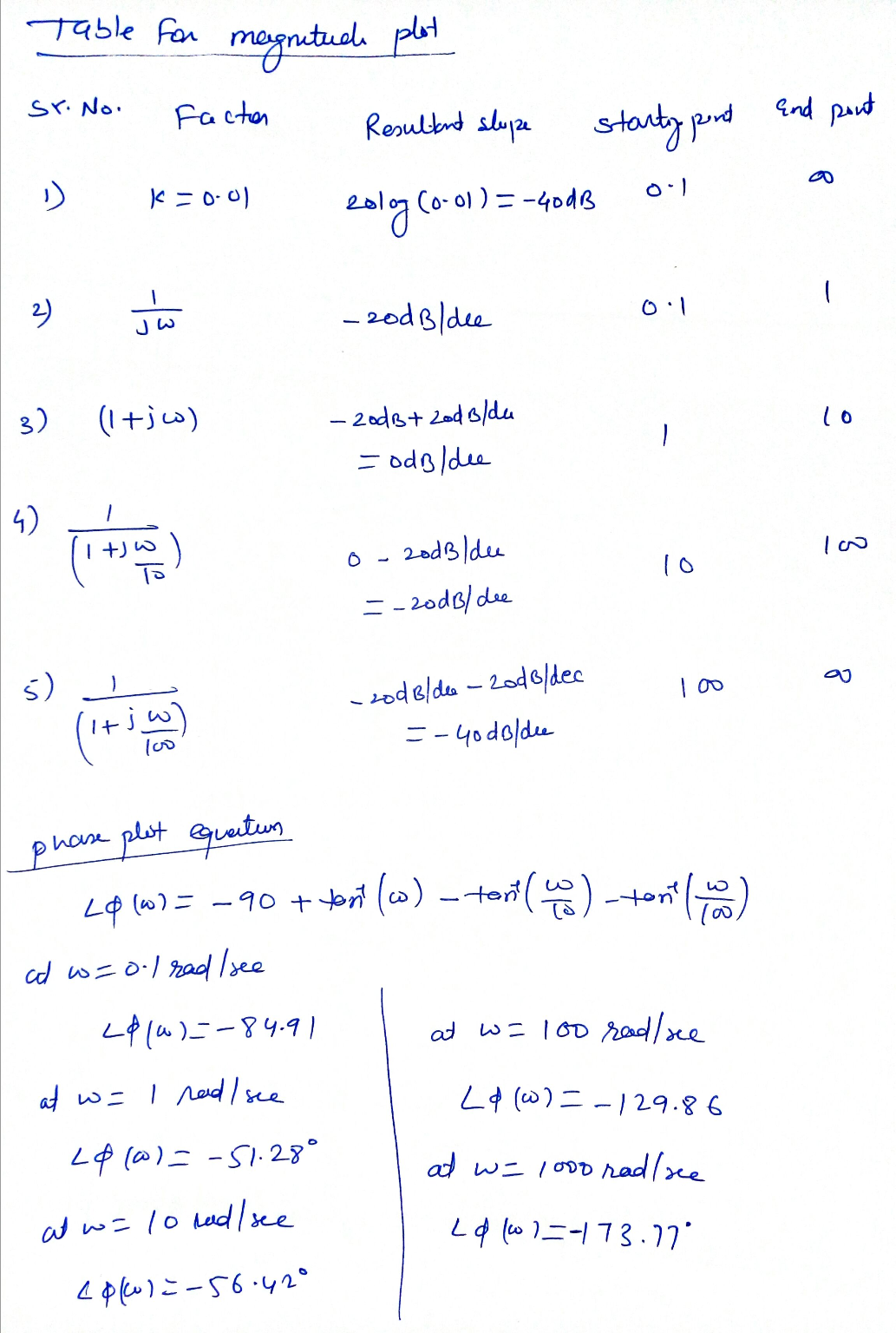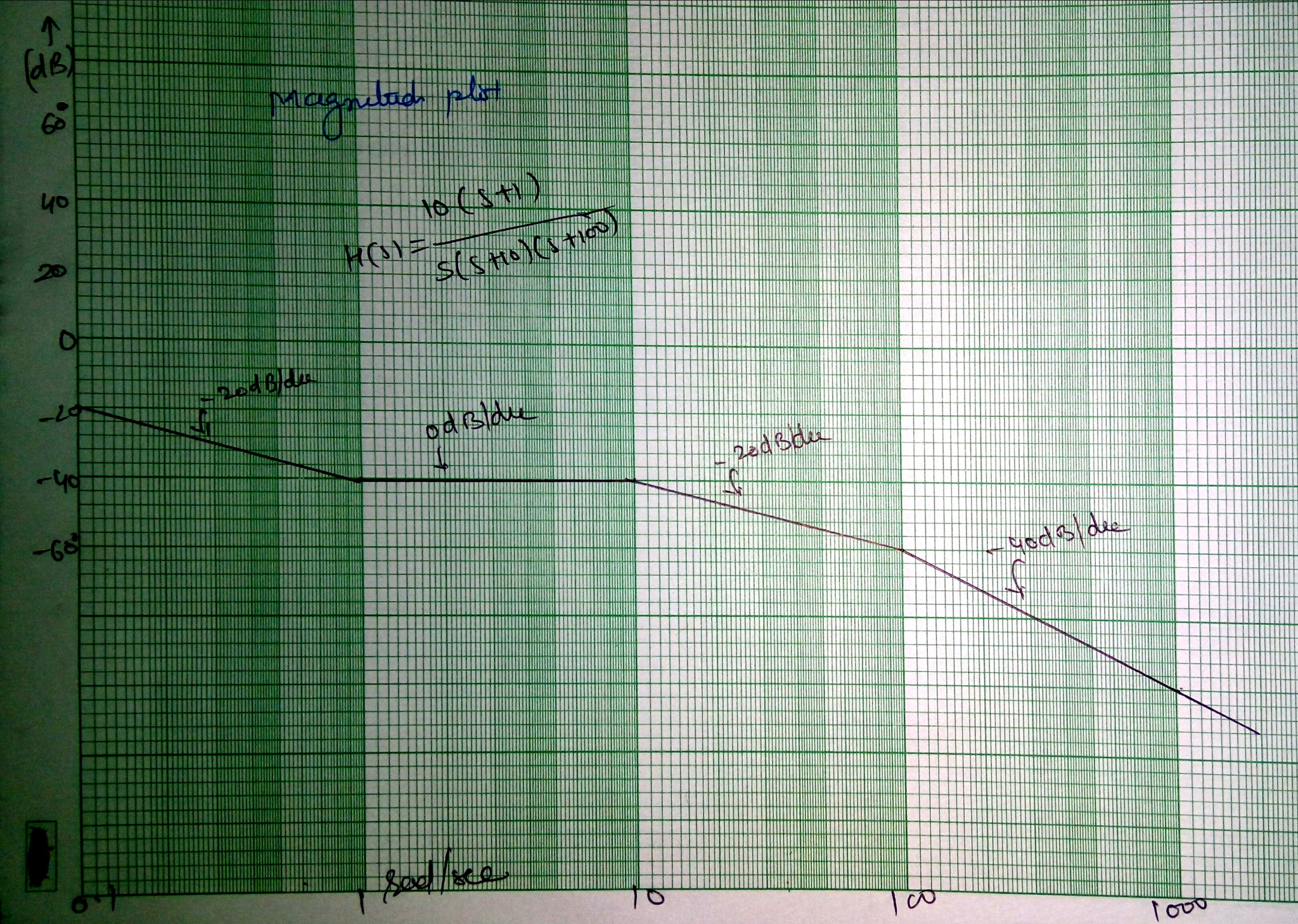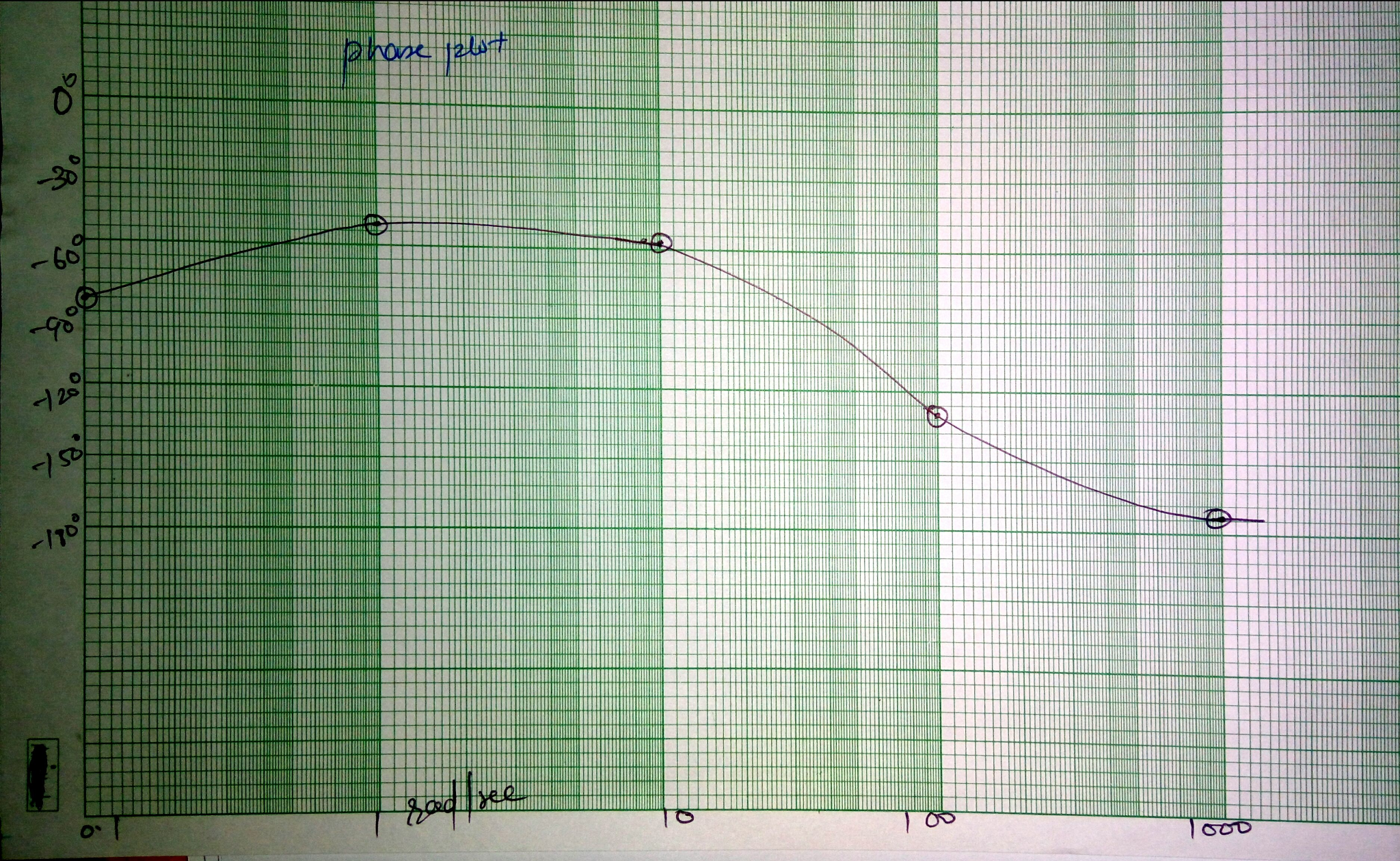Note- please find me in comment box if you find any difficulty. Good luck

#### Earn Coins

Coins can be redeemed for fabulous gifts.

Similar Homework Help Questions
• ### Bode Plots Sketch the Bode plot magnitude and phase for each of the three open-loop transfer...

Bode Plots Sketch the Bode plot magnitude and phase for each of the three open-loop transfer functions listed below. Verify your results using the bode m function in MATLAB.(a) $$G(s)=\frac{100}{s(0.1 s+1)(0.01 s+1)}$$(b) $$G(s)=\frac{1}{(s+1)^{2}\left(s^{2}+s+9\right)}$$(c) $$G(s)=\frac{16000 s}{(s+1)(s+100)\left(s^{2}+5 s+1600\right)}$$

• ### 25 G(s) draw the bode (magnitude and  For the system with transfer function s2+4s+25 phase) plot on the semi-log pa...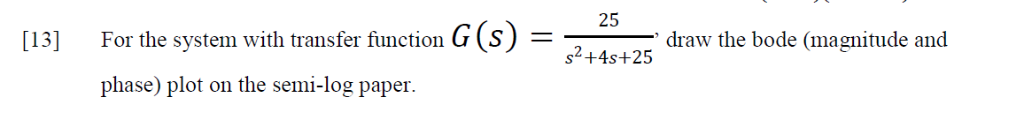25 G(s) draw the bode (magnitude and  For the system with transfer function s2+4s+25 phase) plot on the semi-log paper 25 G(s) draw the bode (magnitude and  For the system with transfer function s2+4s+25 phase) plot on the semi-log paper

• ### i) Draw the Bode plots (hand sketch, magnitude and phase!) for the following transfer function. Plot...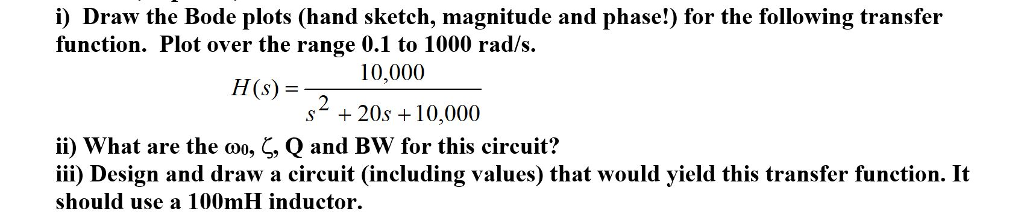i) Draw the Bode plots (hand sketch, magnitude and phase!) for the following transfer function. Plot over the range 0.1 to 1000 rad/s HS 10,000 (s) = s* + 20s 10,000 ii) what are the Q and Bw for this circuit? iii) Design and draw a circuit (including values) that would yield this transfer function. It should use a 100mH inductor , , Qano

• ### For the following transfer function, sketch approximate straight-line Bode plots, including magnitude and phase plots. Show...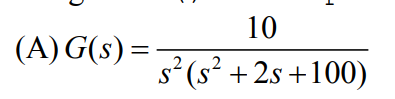For the following transfer function, sketch approximate straight-line Bode plots, including magnitude and phase plots. Show all steps clearly 10 4 (A)G(s)-7 s (s 2s +100)

• ### Steps would be much appreciated A.) Sketch each terms magnitude and phase. B.) Plot the bode...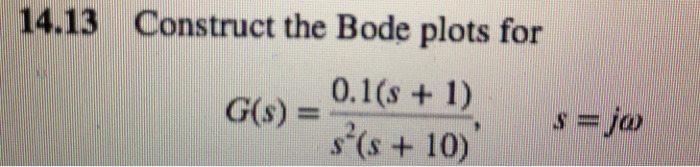Steps would be much appreciated A.) Sketch each terms magnitude and phase. B.) Plot the bode plot for the function. 14.13 Construct the Bode plots for Gs) 0.1s + D s(s +10) ja

• ### For the transfer function, construct the bode plots for magnitude and phase. Use copies of the...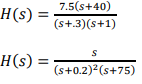For the transfer function, construct the bode plots for magnitude and phase. Use copies of the semi-log paper on the second page of this assignment. Also, a printable copy of the semi-log paper can be found below. Use one sheet per problem. Indicate the contributions from the factors of the transfer function with light lines in pencil. These are called “guidelines”. Indicate the graphical sum of the guidelines (the final bode plot) with heavier lines in ink. You will find...

• ### Sketch the approximate Bode magnitude and phase plots for the following transfer functions by hand. a....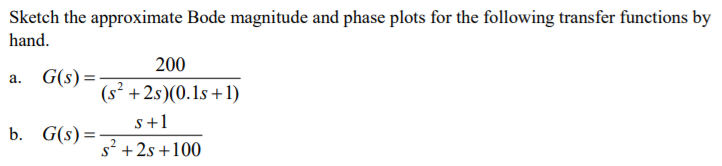Sketch the approximate Bode magnitude and phase plots for the following transfer functions by hand. a. G(s) b. G(s)- 200 (s2 +2s)(0.1s +1) s+1 s2 +2s +100

• ### Q5) Sketch the Bode Plot (magnitude response) of the following systems. NOTE: Try to sketch yourself...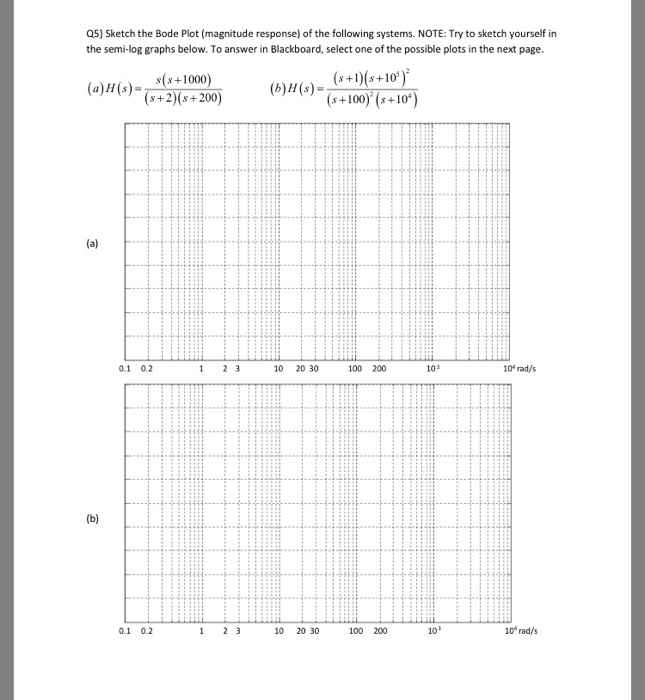Q5) Sketch the Bode Plot (magnitude response) of the following systems. NOTE: Try to sketch yourself in the semi-log graphs below. To answer in Blackboard, select one of the possible plots in the next page (a)H(+1000 s+2) (s+200) +100) (+10) 0.1 02 10 20 30 100 200 10 rad/s 0.1 0.2 10 20 30 100 200 10 rad/s

• ### Sketch the straight-line approximation Bode plot diagrams (magnitude and phase) 110s for H[s] You might want...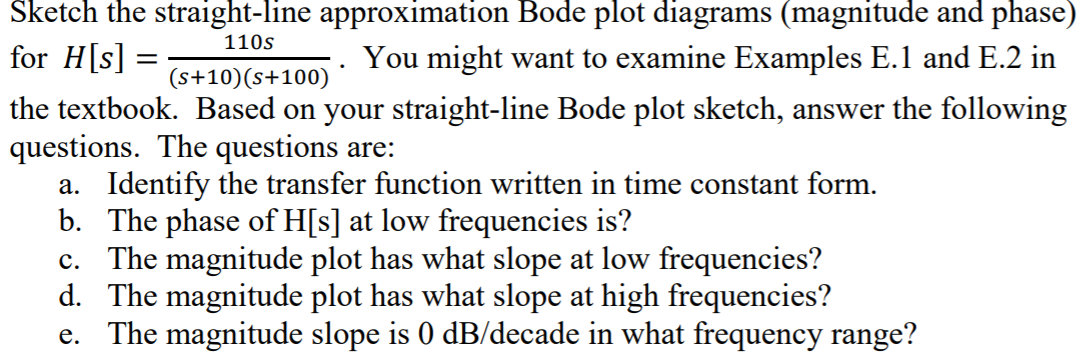Sketch the straight-line approximation Bode plot diagrams (magnitude and phase) 110s for H[s] You might want to examine Examples E.1 and E.2 in (s+10)(s+100)´ the textbook. Based on your straight-line Bode plot sketch, answer the following questions. The questions are: a. Identify the transfer function written in time constant form. b. The phase of H[s] at low frequencies is? c. The magnitude plot has what slope at low frequencies? d. The magnitude plot has what slope at high frequencies? e....

• ### For the following closed-loop transfer functions, sketch the bode plots (magnitude and phase), id...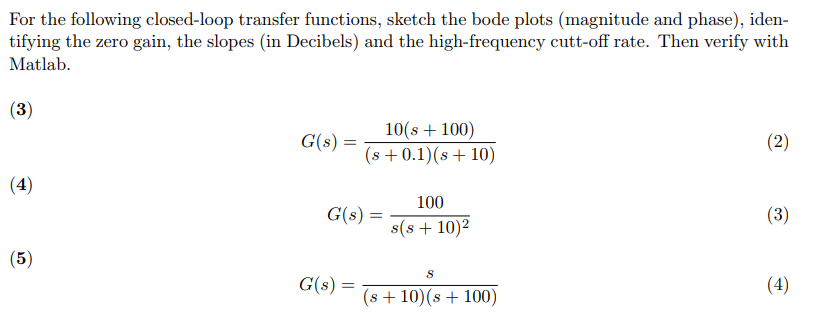For the following closed-loop transfer functions, sketch the bode plots (magnitude and phase), iden- tifying the zero gain, the slopes (in Decibels) and the high-frequency cutt-off rate. Then verify with Matlab C()101 100) s 0.1) (s 10) 100 s(s +10)2 G(s) = (56) G(s) = s+10(s+100) For the following closed-loop transfer functions, sketch the bode plots (magnitude and phase), iden- tifying the zero gain, the slopes (in Decibels) and the high-frequency cutt-off rate. Then verify with Matlab C()101 100) s...